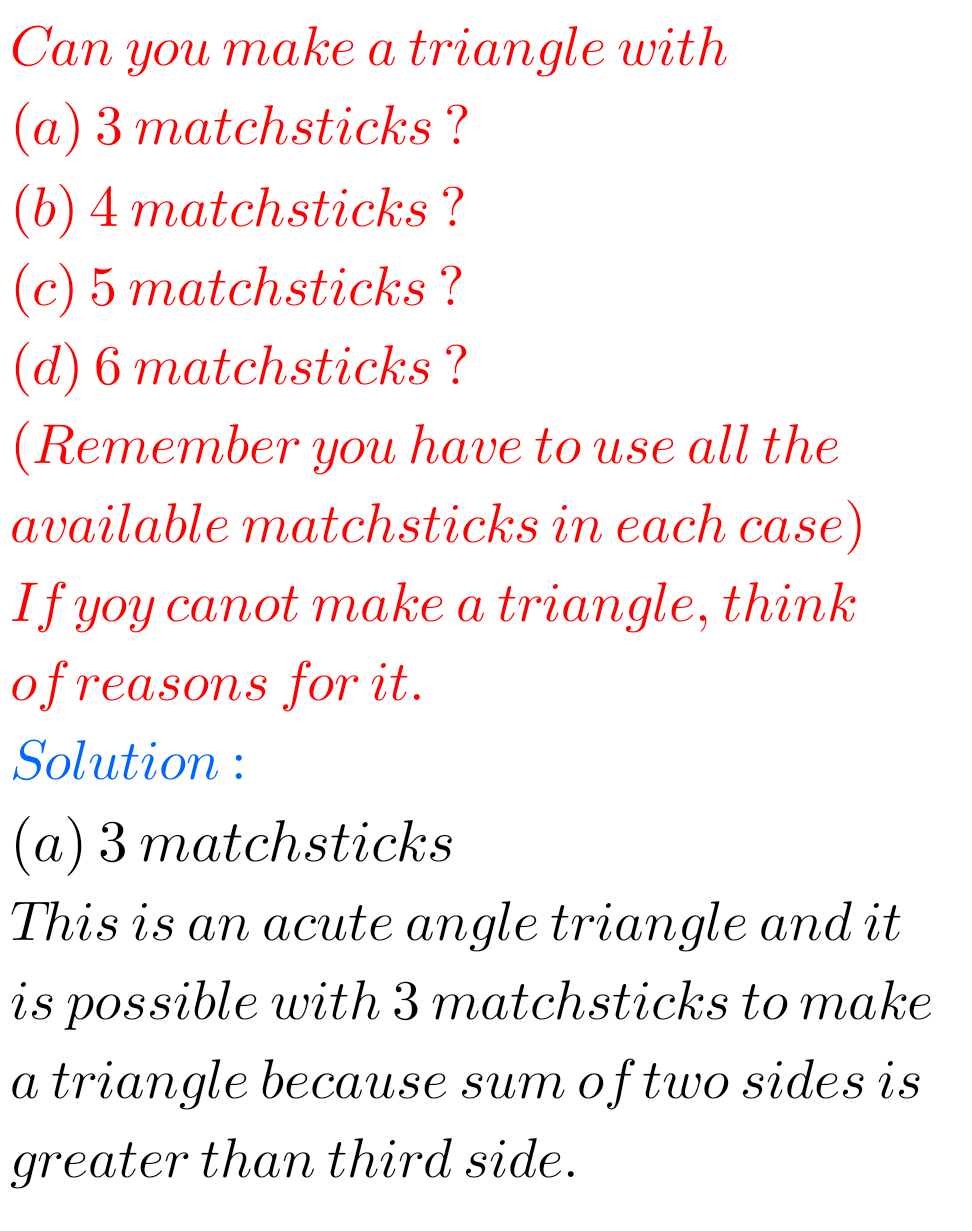## Solutions for exercise 5.6 chapter 5 class 6 Maths Ncert

Sixth class Maths Understanding Elementary Shapes exercise 5.6 chapter 5 solutions Ncert Ncert Maths Class 6 chapter 5 Understanding Elementary Shapes Exercise 5.6 solutions are given. Study the textbook lesson Understanding Elementary Shapes very well. Observe the example problems and solutions given in the textbook. Observe the given solutons and try them in your own …